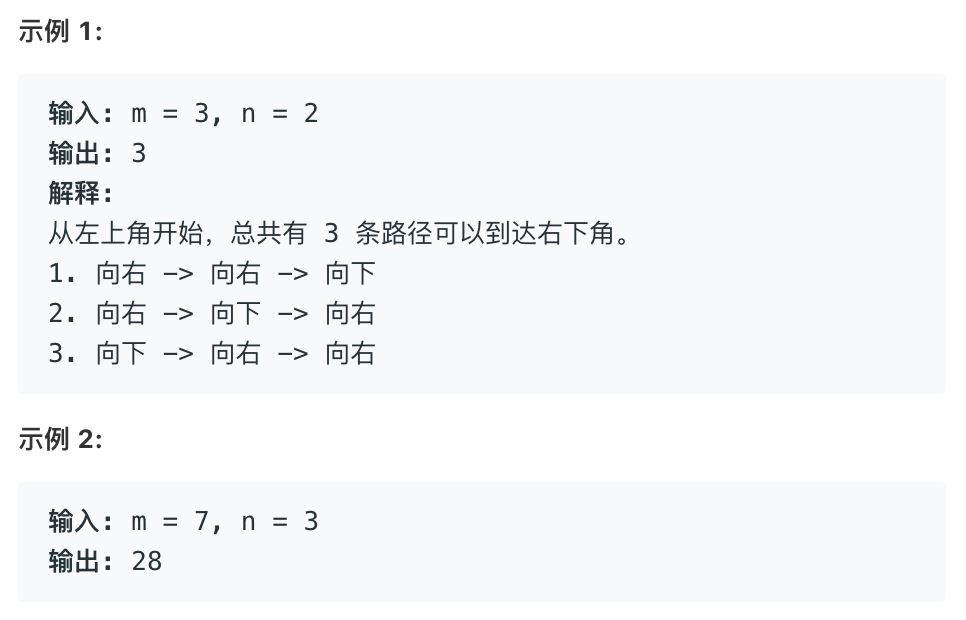# 62. 不同路径

2019-05-14 16:39 190 查看# 解1 递归

dfs深度优先遍历

```class Solution:
def uniquePaths(self, m: int, n: int) -> int:

result = list()
self.dfs(m - 1, n - 1, result)
return sum(result)

def dfs(self, m, n, result):

if m == 0 or n == 0:
result.append(1)

else:
self.dfs(m - 1, n, result)
self.dfs(m, n - 1, result)```

# 解2 找规律

```class Solution:
def uniquePaths(self, m: int, n: int) -> int:

# 向右走的步数
r = m - 1
# 向左走的步数
l = n - 1

# 总共走的步数
s = r + l

# s步中选r步向右走 或 选l步向左走
a = 1
b = 1

if r < l:

for i in range(r):
a *= s
b *= r
s -= 1
r -= 1
return int(a / b)

else:

for i in range(l):
a *= s
b *= l
s -= 1
l -= 1
return int(a / b)```

# 解3 动态规划

path[i][j] 表示从左上角到达 i j 方格总共有多少条路径，则

1. path[j] == path[i] == 1 上边和左边都只有一条路径
2. path[i][j] = path[i - 1][j] + path[i][j - 1] 到达上边方格的路径数 + 到达左边方格的路径数
```class Solution:
def uniquePaths(self, m: int, n: int) -> int:

path = [[1 for i in range(n)] for j in range(m)]

for i in range(1, m):
for j in range(1, n):
path[i][j] = path[i - 1][j] + path[i][j - 1]

return path[m - 1][n - 1]```